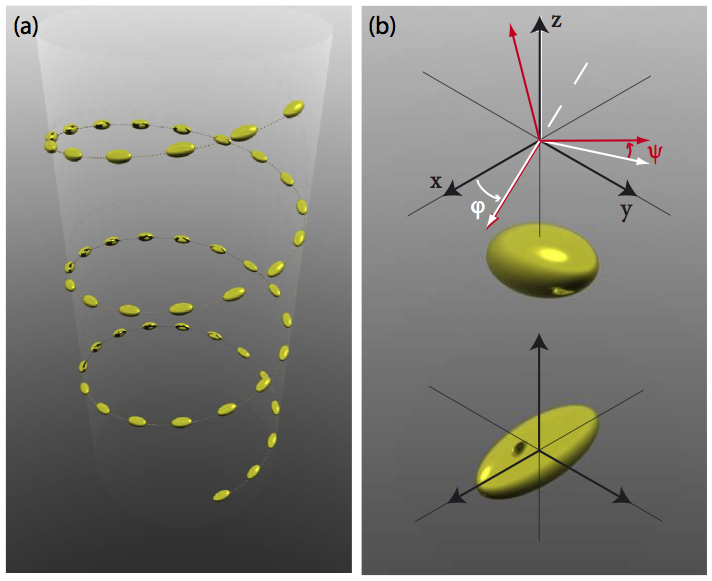# Circular dichroism in assemblies of plasmonic nanoparticles – modelling in the dipole approximation

The cda package implements the coupled-dipole approximation for electromagnetic scattering by sparse collections of subwavelength particles, with a particular focus on plasmonic nanoparticles in the visible regime. The interaction matrix is formed in C++ code for speed; convenient wrapper functions are provided at the R level to calculate the extinction, scattering, and absorption of light by particles with linearly and circularly polarised light. Functions are also provided to calculate orientation-averaged circular dichroism, and display clusters of nanoparticles in three dimensions using RGL or povray.

The system consists in a three-dimensional arrangement of small ellipsoidal particles in arbitrary orientations, as shown in figure 1. Here the position and orientation of the particles was chosen to follow a helix.## Coupled dipole model

Each dipole scatters light in proportion to the local field it experiences,

${\mathbf{p}_{\text{dip}}}=\alpha {\mathbf{E}_{\text{loc}}}, \label{eq:localfield}$

where $$\alpha$$ is the polarizability tensor describing the individual nanoparticle. The prescription of Kuwata et al. (Appl. Phys. Lett. 83, 4625 (2003)) was chosen, which provides an accurate approximation for particles below $\sim 100$nm in size. Each dipole may be placed in arbitrary orientation, and to this end the polarizability tensor must be transformed to a rotated frame.

Let us describe each nanoparticle by a diagonal polarizability tensor in the reference frame of its principal axes. The rotation of this reference frame to the actual position of the particle can be described by three Euler angles $$\phi, \theta, \psi$$ and a rotation matrix 

$\mathrm{R}= \begin{bmatrix} \cos \psi \cos \phi - \cos \theta \sin \phi \sin \psi & \cos \psi \sin \phi + \cos \theta \cos \phi \sin \psi & \sin \psi \sin \theta \ -\sin \psi \cos \phi - \cos \theta \sin \phi \cos \psi & -\sin \psi \sin \phi + \cos \theta \cos \phi \cos \psi & \cos \psi \sin \theta \ \sin \phi \sin \theta & -\cos \phi \sin \theta & \cos \theta \end{bmatrix}. \label{eq:euler}$

A dipole in orientation $$\phi, \theta, \psi$$ will be described by a polarizability $$\mathrm{R}^{-1}\alpha \mathrm{R}$$ in the global reference frame.

The local field

${\mathbf{E}_{\text{loc}}}={\mathbf{E}_{\text{inc}}}+\sum_{\text{dipoles}\setminus \text{itself}}{{\mathbf{E}_{\text{d}}}}, \label{eq:dda}$

is the sum of the incident field plus the contribution of the dipolar field associated with the other dipoles in the system. The field radiated by a dipole reads,

${\mathbf{E}_{\text{d}}}=\frac{e^{i\omega r/c}}{4\pi\varepsilon_0}\left\{\frac{\omega^2}{c^2r}\mathbf{\hat r}\times{\mathbf{p}}\times\mathbf{\hat r}+\left(\frac{1}{r^3}-\frac{i\omega}{cr^2}\right)\left[3(\mathbf{\hat r}\cdot{\mathbf{p}})\mathbf{\hat r}-{\mathbf{p}}\right]\right\}. \label{eq:dipoleField}$

By grouping together the dipole moments we can cast equation [eq:dda] in matrix form,

$A{\mathbf{P}}={\mathrm{E}_{\text{inc}}}, \label{eq:DDAmatrix}$

where $$A$$ is the interaction matrix that describes the electromagnetic coupling between the dipoles in the non-diagonal blocks,

$A_{ij}=\dfrac{e^{(ikr_{ij})}}{r_{ij}}\left\{k^2(\mathbf{\hat r}\otimes\mathbf{\hat r}-{\mathbb I})+\dfrac{ikr_{ij}-1}{r_{ij}^2}(3\mathbf{\hat r}\otimes\mathbf{\hat r}-{\mathbb I})\right\}, \label{eq:interactionMatrix}$

and the block diagonal $$A_{ii}=\alpha^{-1}$$ is formed with the inverse polarizability of the individual dipoles, in the global $$(x,y,z)$$ reference frame.

When the dipole moments are known by inversion of equation [eq:DDAmatrix], the extinction cross-section can be obtained for a given incident field following,

$\sigma_{\rm ext}=\frac{4\pi k}{|{\mathrm{E}_{\text{inc}}}|^2}\Im({\mathbf{E}_{\text{inc}}}^*\cdot {\mathbf{P}}). \label{eq:extinctionDDA}$

## Computation of circular dichroism

Circular dichroism can be calculated from the difference in extinction for left-handed and right-handed circularly polarised light, averaged over the full solid angle of incident light,

$\sigma_{\text{CD}} = \left<\sigma_L\right>_\Omega - \left<\sigma_R\right>_\Omega.$

The incident field incident along $$x$$ is written as,

\begin{aligned} {\mathbf{E}_{\text{inc}}}&= \frac{\exp i(\omega t - k_xx)}{\sqrt{2}} \begin{pmatrix} 0\ i\ 1 \end{pmatrix}\quad (\text{right-handed})\ {\mathbf{E}_{\text{inc}}}&= \frac{\exp i(\omega t - k_xx)}{\sqrt{2}} \begin{pmatrix} 0\ 1\ i \end{pmatrix} \quad (\text{left-handed}).\end{aligned}

More generally, the incident beam will be characterised by a wave-vector $$\mathbf{k}$$ and an electric vector $${\mathbf{E}_{\text{inc}}}$$ describing the light polarisation, and both of these vectors can be rotated using the rotation matrix $$R$$ as $$\mathrm{R}^{-1}\mathbf{k}$$ and $$\mathrm{R}^{-1}{\mathbf{E}_{\text{inc}}}$$.

The interaction matrix $$A$$ does not depend on the direction of the incident field, it may therefore be advantageous to compute $$A^{-1}$$ (at each wavelength) and perform the matrix-vector products for all the required incident fields. The CD spectra obtained experimentally are averaged over all orientations of the incident beam, it is therefore necessary to use incident wave-vectors that span the full range of $$\phi\in[0,2\pi], \theta=\pi/2, \psi\in[-\pi/2,\pi/2]$$.

Averaging the extinction cross-section over all incident field directions is performed by numerical integration,

$\left<\sigma\right>_\Omega=\frac1 {4\pi} \int_0^{2\pi}\int_{-\pi/2}^{\pi/2} \sigma(\phi,\psi) \cos \psi d\psi d\phi.$

A Gauss-Legendre quadrature scheme is used to perform the integration, so that the evaluation of the extinction cross-section is performed for a relatively low number of angles ($$20\times 20$$ seems sufficient). Other integration schemes are also possible.

: using the same conventions as http://mathworld.wolfram.com/EulerAngles.html